# Selina Solutions Concise Mathematics Class 6 Chapter 24 Angles Exercise 24(B)

Selina Solutions Concise Mathematics Class 6 Chapter 24 Angles Exercise 24(B) has answers curated by expert faculty at BYJU’S, which are 100% accurate. This exercise deals with the study of concepts relying on complementary and supplementary angles in detail. The solutions, designed by experts in simple language improve logical thinking and problem solving skills among students. By referring to these solutions, students obtain conceptual knowledge about the concepts. To clear their doubts immediately, students can use Selina Solutions Concise Mathematics Class 6 Chapter 24 Exercise 24(B) PDF, from the links given below.

## Selina Solutions Concise Mathematics Class 6 Chapter 24: Angles Exercise 24(B) Download PDF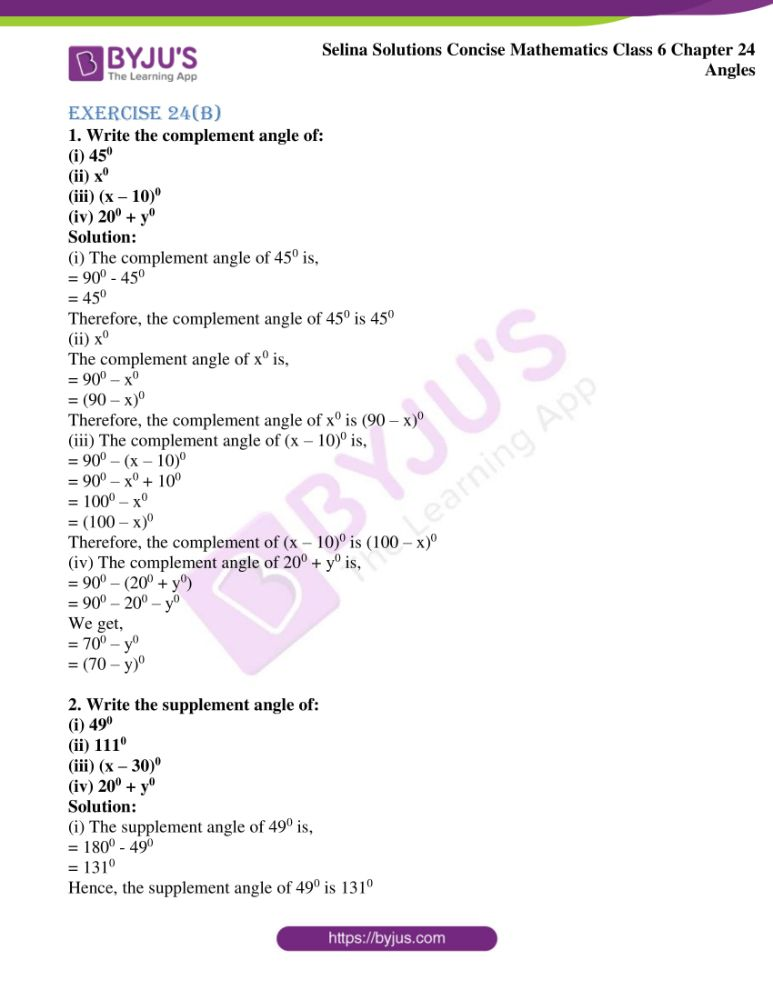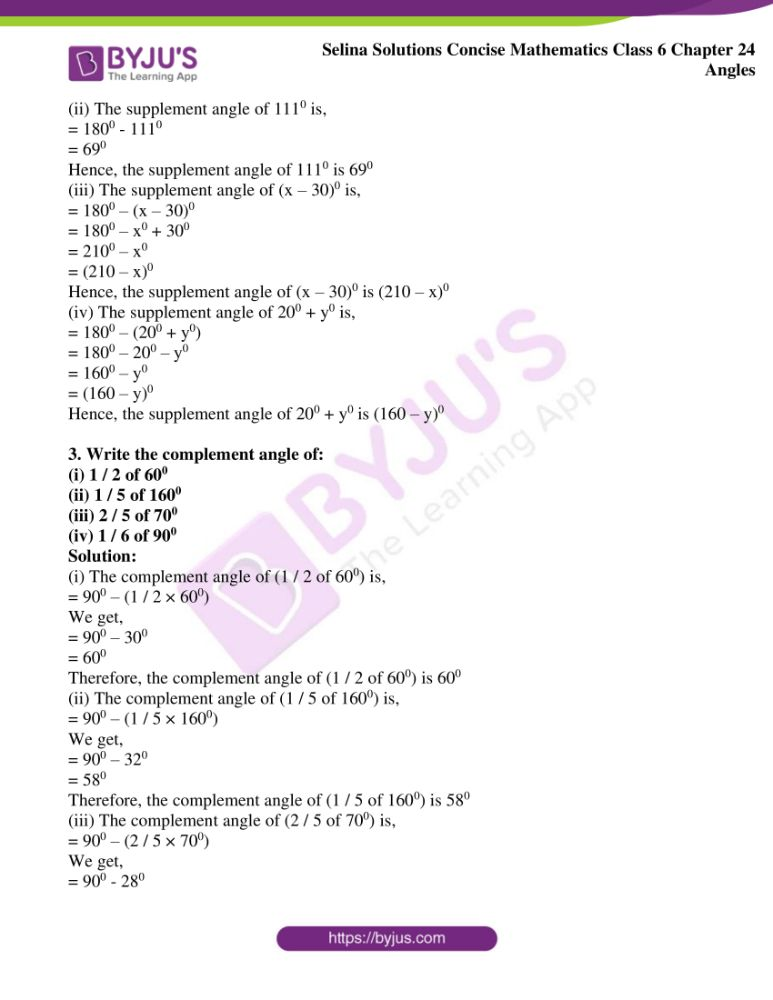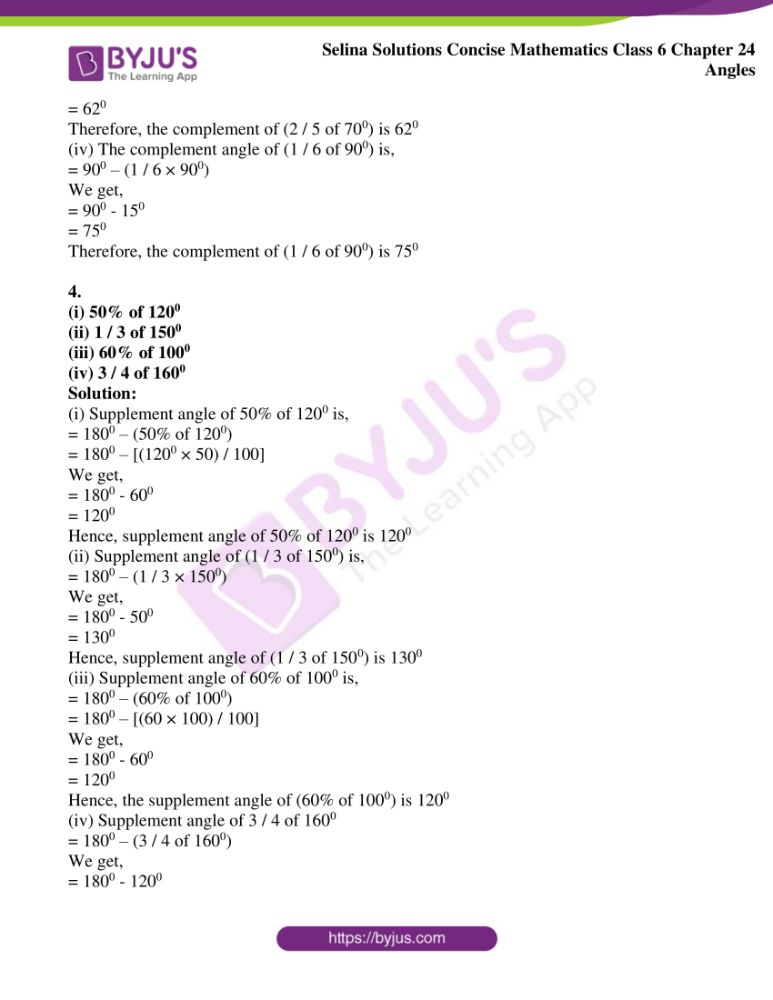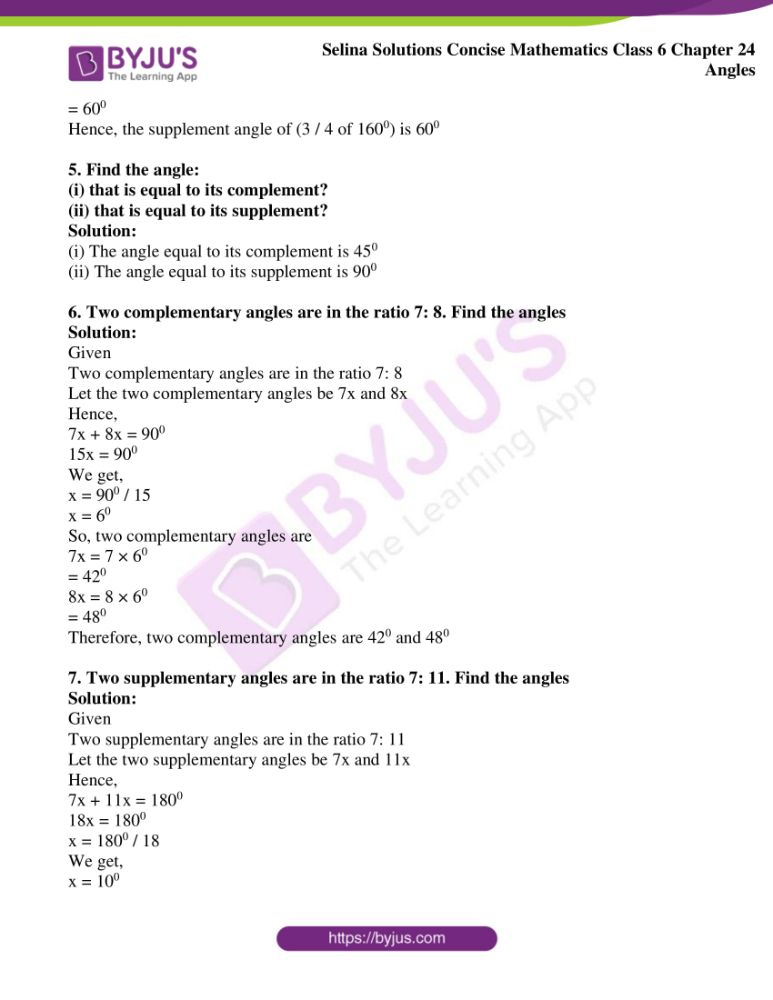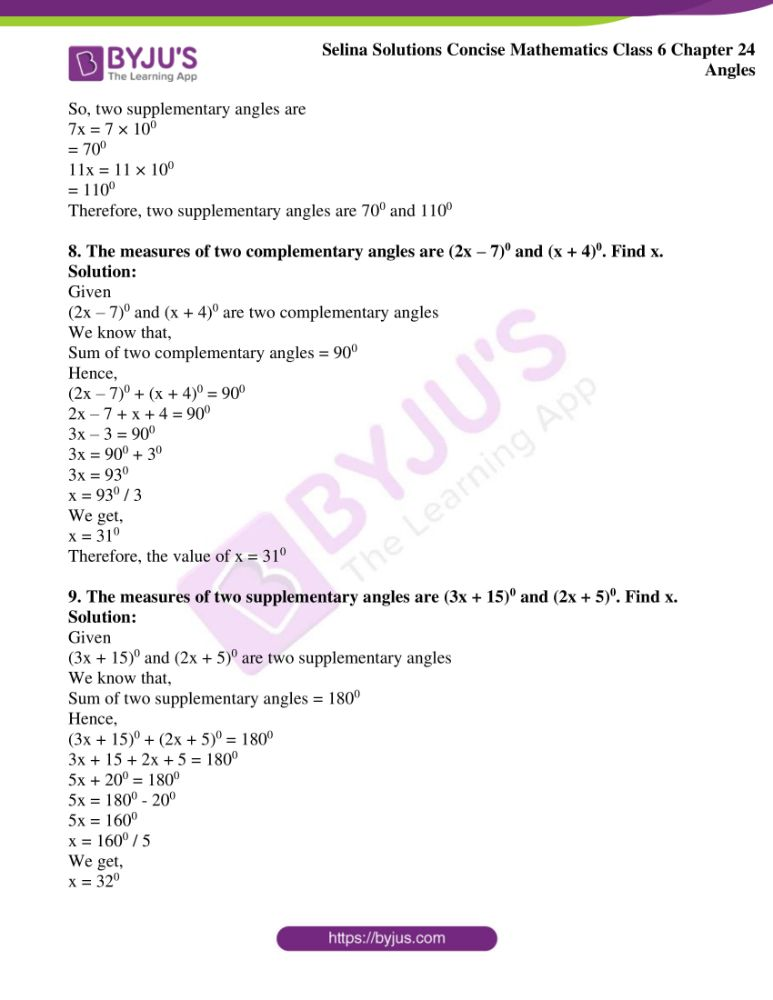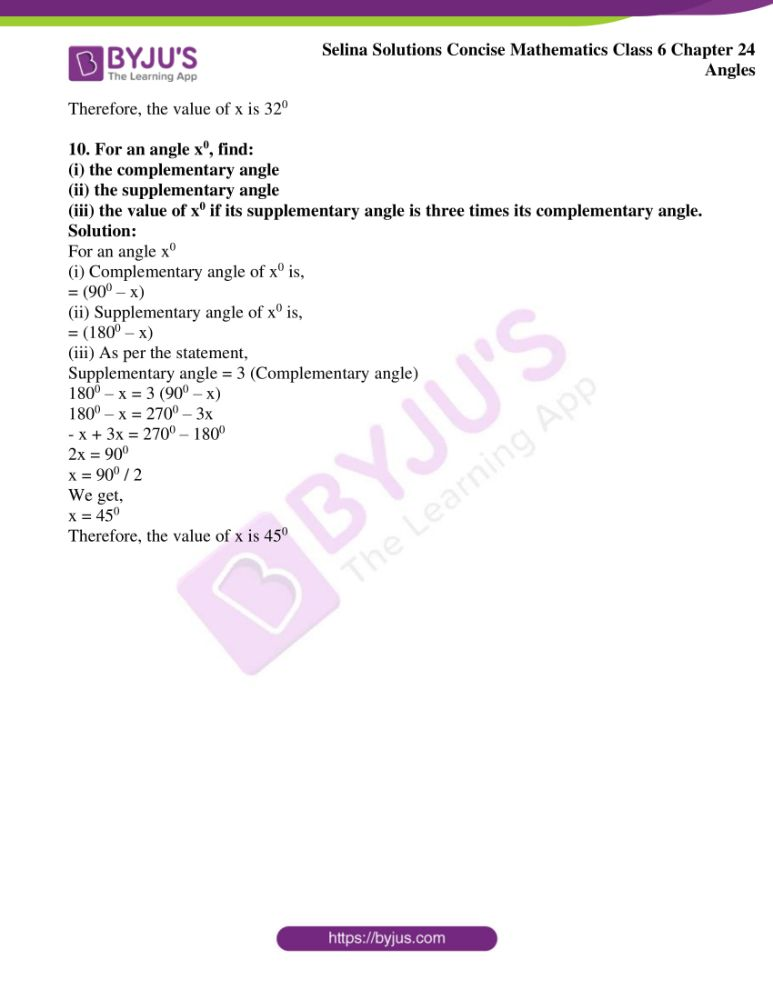### Access Selina Solutions Concise Mathematics Class 6 Chapter 24: Angles Exercise 24(B)

1. Write the complement angle of:

(i) 450

(ii) x0

(iii) (x – 10)0

(iv) 200 + y0

Solution:

(i) The complement angle of 450 is,

= 900 – 450

= 450

Therefore, the complement angle of 450 is 450

(ii) x0

The complement angle of x0 is,

= 900 – x0

= (90 – x)0

Therefore, the complement angle of x0 is (90 – x)0

(iii) The complement angle of (x – 10)0 is,

= 900 – (x – 10)0

= 900 – x0 + 100

= 1000 – x0

= (100 – x)0

Therefore, the complement of (x – 10)0 is (100 – x)0

(iv) The complement angle of 200 + y0 is,

= 900 – (200 + y0)

= 900 – 200 – y0

We get,

= 700 – y0

= (70 – y)0

2. Write the supplement angle of:

(i) 490

(ii) 1110

(iii) (x – 30)0

(iv) 200 + y0

Solution:

(i) The supplement angle of 490 is,

= 1800 – 490

= 1310

Hence, the supplement angle of 490 is 1310

(ii) The supplement angle of 1110 is,

= 1800 – 1110

= 690

Hence, the supplement angle of 1110 is 690

(iii) The supplement angle of (x – 30)0 is,

= 1800 – (x – 30)0

= 1800 – x0 + 300

= 2100 – x0

= (210 – x)0

Hence, the supplement angle of (x – 30)0 is (210 – x)0

(iv) The supplement angle of 200 + y0 is,

= 1800 – (200 + y0)

= 1800 – 200 – y0

= 1600 – y0

= (160 – y)0

Hence, the supplement angle of 200 + y0 is (160 – y)0

3. Write the complement angle of:

(i) 1 / 2 of 600

(ii) 1 / 5 of 1600

(iii) 2 / 5 of 700

(iv) 1 / 6 of 900

Solution:

(i) The complement angle of (1 / 2 of 600) is,

= 900 – (1 / 2 × 600)

We get,

= 900 – 300

= 600

Therefore, the complement angle of (1 / 2 of 600) is 600

(ii) The complement angle of (1 / 5 of 1600) is,

= 900 – (1 / 5 × 1600)

We get,

= 900 – 320

= 580

Therefore, the complement angle of (1 / 5 of 1600) is 580

(iii) The complement angle of (2 / 5 of 700) is,

= 900 – (2 / 5 × 700)

We get,

= 900 – 280

= 620

Therefore, the complement of (2 / 5 of 700) is 620

(iv) The complement angle of (1 / 6 of 900) is,

= 900 – (1 / 6 × 900)

We get,

= 900 – 150

= 750

Therefore, the complement of (1 / 6 of 900) is 750

4.

(i) 50% of 1200

(ii) 1 / 3 of 1500

(iii) 60% of 1000

(iv) 3 / 4 of 1600

Solution:

(i) Supplement angle of 50% of 1200 is,

= 1800 – (50% of 1200)

= 1800 – [(1200 × 50) / 100]

We get,

= 1800 – 600

= 1200

Hence, supplement angle of 50% of 1200 is 1200

(ii) Supplement angle of (1 / 3 of 1500) is,

= 1800 – (1 / 3 × 1500)

We get,

= 1800 – 500

= 1300

Hence, supplement angle of (1 / 3 of 1500) is 1300

(iii) Supplement angle of 60% of 1000 is,

= 1800 – (60% of 1000)

= 1800 – [(60 × 100) / 100]

We get,

= 1800 – 600

= 1200

Hence, the supplement angle of (60% of 1000) is 1200

(iv) Supplement angle of 3 / 4 of 1600

= 1800 – (3 / 4 of 1600)

We get,

= 1800 – 1200

= 600

Hence, the supplement angle of (3 / 4 of 1600) is 600

5. Find the angle:

(i) that is equal to its complement?

(ii) that is equal to its supplement?

Solution:

(i) The angle equal to its complement is 450

(ii) The angle equal to its supplement is 900

6. Two complementary angles are in the ratio 7: 8. Find the angles

Solution:

Given

Two complementary angles are in the ratio 7: 8

Let the two complementary angles be 7x and 8x

Hence,

7x + 8x = 900

15x = 900

We get,

x = 900 / 15

x = 60

So, two complementary angles are

7x = 7 × 60

= 420

8x = 8 × 60

= 480

Therefore, two complementary angles are 420 and 480

7. Two supplementary angles are in the ratio 7: 11. Find the angles

Solution:

Given

Two supplementary angles are in the ratio 7: 11

Let the two supplementary angles be 7x and 11x

Hence,

7x + 11x = 1800

18x = 1800

x = 1800 / 18

We get,

x = 100

So, two supplementary angles are

7x = 7 × 100

= 700

11x = 11 × 100

= 1100

Therefore, two supplementary angles are 700 and 1100

8. The measures of two complementary angles are (2x – 7)0 and (x + 4)0. Find x.

Solution:

Given

(2x – 7)0 and (x + 4)0 are two complementary angles

We know that,

Sum of two complementary angles = 900

Hence,

(2x – 7)0 + (x + 4)0 = 900

2x – 7 + x + 4 = 900

3x – 3 = 900

3x = 900 + 30

3x = 930

x = 930 / 3

We get,

x = 310

Therefore, the value of x = 310

9. The measures of two supplementary angles are (3x + 15)0 and (2x + 5)0. Find x.

Solution:

Given

(3x + 15)0 and (2x + 5)0 are two supplementary angles

We know that,

Sum of two supplementary angles = 1800

Hence,

(3x + 15)0 + (2x + 5)0 = 1800

3x + 15 + 2x + 5 = 1800

5x + 200 = 1800

5x = 1800 – 200

5x = 1600

x = 1600 / 5

We get,

x = 320

Therefore, the value of x is 320

10. For an angle x0, find:

(i) the complementary angle

(ii) the supplementary angle

(iii) the value of x0 if its supplementary angle is three times its complementary angle.

Solution:

For an angle x0

(i) Complementary angle of x0 is,

= (900 – x)

(ii) Supplementary angle of x0 is,

= (1800 – x)

(iii) As per the statement,

Supplementary angle = 3 (Complementary angle)

1800 – x = 3 (900 – x)

1800 – x = 2700 – 3x

– x + 3x = 2700 – 1800

2x = 900

x = 900 / 2

We get,

x = 450

Therefore, the value of x is 450